图像处理中dct是_dct图像处理 - CSDN
• DCT变换，也就是离散余弦变换（Discrete Cosine Transform)是图像频域变换的一种，实际上可以看成是一种空域的低通...目前，离散余弦变换以及它的改进算法已经成为广泛应用于信号处理和图像处理，特别是用于图像压缩
DCT变换，也就是离散余弦变换（Discrete Cosine Transform)是图像频域变换的一种，实际上可以看成是一种空域的低通滤波器，DCT也可以看做是傅里叶变换的一种特殊情况。在傅里叶级数中，如果被展开的函数是实偶函数，那么在傅里叶级数中则只包含余弦项，再将其离散化，由此便可导出离散余弦变化。
目前，离散余弦变换以及它的改进算法已经成为广泛应用于信号处理和图像处理，特别是用于图像压缩和语音压缩编解码的重要工具和技术。这是由于DCT具有很强的“能量集中”的特性，大多数的自然信号（包括声音和图像）的能量都集中在离散余弦变换后的低频部分，DCT的作用是吧图像中点和点间的规律呈现出来，虽然DCT本身并没有压缩作用，但是却为以后压缩时的“取舍”奠定了必不可少的基础。
离散余弦变换在某种程度上与离散傅里叶变换有写类似，但是与DFT不同的是DCT只使用实数部分，DFT需要计算的是复数而非实数，而进行复数运算通常比计算实数运算费时得多，所以DCT相当于一个长度是其二被的DFT，从形式上看，DCT是一个线性的可逆函数。
下面先简单介绍一下DCT的数学基础
一维的DCT的正变换公式如下：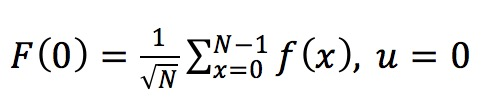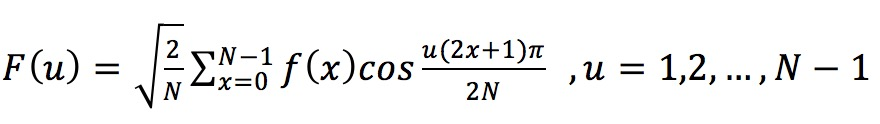其中，F(u)是第u个DCT变换的系数，f(x)是时域中的N点的序列，x=0,1,2...,N-1
二维图像的DCT变换形式为：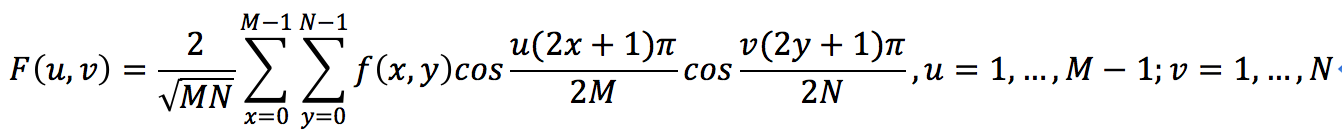其中，f(x,y)为空域中的二维向量，x=0,1,2....M-1;y = 0,1,2...,N-1;F(u,v)为变换系数矩阵
使用OpenCV进行简单的实现程序如下：

//实现二维图像的DCT

#include <iostream>
#include <opencv2\core\core.hpp>
#include <opencv2\highgui\highgui.hpp>
#include <opencv2\imgproc\imgproc.hpp>

using namespace cv;
using namespace std;

int main()
{

if (!srcImage.data)
{
cout << "读入图像出错" << endl;
return -1;
}
imshow("原图像", srcImage);
int height = srcImage.rows;
int width = srcImage.cols;

//从BGR空间转换到YUV颜色空间
Mat yuvImage(srcImage.size(), CV_8UC3);
cvtColor(srcImage, yuvImage, CV_BGR2YUV);
//定义输出图像
Mat dstImage(srcImage.size(), CV_64FC3);

//分割YUV三个通道
vector<Mat> channels;
split(yuvImage, channels);

//提取YUV颜色各个通道的值
Mat y = channels.at(0);	imshow("Y", y);
Mat u = channels.at(1); imshow("U", u);
Mat v = channels.at(2); imshow("V", v);

//定义DCT系数的三个通道
Mat DCTY(srcImage.size(), CV_64FC1);
Mat DCTU(srcImage.size(), CV_64FC1);
Mat DCTV(srcImage.size(), CV_64FC1);

//进行DCT变换
dct(Mat_<double>(y), DCTY);
dct(Mat_<double>(u), DCTU);
dct(Mat_<double>(v), DCTV);

channels.at(0) = Mat_<uchar>(DCTY);
channels.at(1) = Mat_<uchar>(DCTU);
channels.at(2) = Mat_<uchar>(DCTV);

merge(channels,dstImage);

imshow("DCT图像", dstImage);

waitKey();
return 0;
}


展开全文• 常用于图像数据的压缩，通过将图像分成大小相等（一般为8*8）的块，利用DCT对其进行变换，得到更加简洁的数据。因为图像像素间存在较大的空间相关性，DCT可以大大减小这些相关性，使图像能量集中在左上角区域

转自：http://blog.csdn.net/ahafg/article/details/48808443
DCT又称离散余弦变换，是一种块变换方式，只使用余弦函数来表达信号，与傅里叶变换紧密相关。常用于图像数据的压缩，通过将图像分成大小相等（一般为8*8）的块，利用DCT对其进行变换，得到更加简洁的数据。因为图像像素间存在较大的空间相关性，DCT可以大大减小这些相关性，使图像能量集中在左上角区域，从而利于数据压缩。变换后得到的数据称为DCT系数。这一过程是无损的。

二维DCT变换
这里来看看二维DCT变换的公式：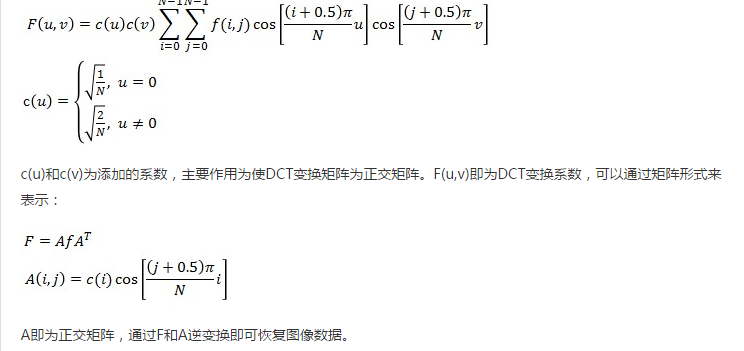clear;
clc;
I = [12,23,53,16;42,16,68,45;34,62,73,26;72,15,34,28];  %数据块
A = zeros(4);   %变换矩阵A,也可以通过函数dctmtx(n)求得
for i = 0:3
for j = 0:3
if i == 0
a = sqrt(1/4);
else
a = sqrt(2/4);
end
A(i+1,j+1) = a*cos((j+0.5)*pi*i/4)
end
end
D = A*I*A';     %DCT变换
D1 = dct2(I);   %matlab DCT函数进行DCT变换
D2 = A'*D*A;    %DCT逆变换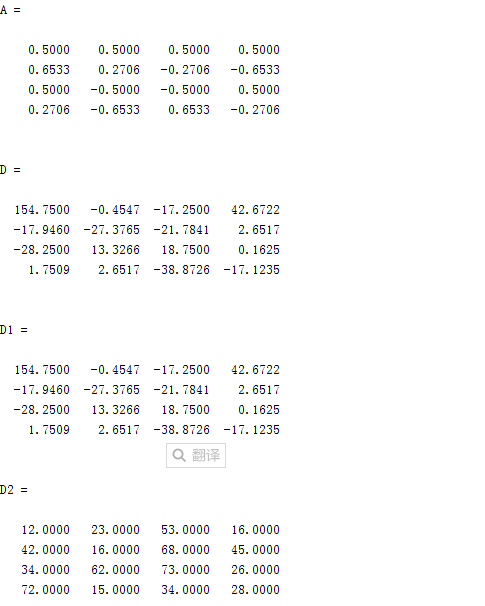由结果可以看出，D，D1方式得到的DCT系数相同，说明矩阵形式的DCT变换公式是正确的，D2的数据与原数据I相同，实现了数据恢复。
另外通过运行函数dctmtx(4)可以发现得到的变换矩阵与A完全相同。
Matlab 函数实现
matlab实现离散余弦变换有两种方法：
一种为函数dct2( ), 使用函数dct2，该函数用一个基于FFT的算法来提高当输入较大的方阵时的计算速度。另一种为函数dctmtx( ), 使用由dctmtx函数返回的DCT变换矩阵，这种方法较适合于较小的输入方阵（例如8×8或16×16）。
. 函数：dct2( )
实现图像的二维离散余弦变换。调用格式为：
B = dct2(A)
B = dct2(A,[M N])
B = dct2(A,M,N)
式中A表示要变换的图像，M和N是可选参数，表示填充后的图像矩阵大小，B表示变换后得到的图像矩阵。其逆变换函数为idct2( );

D = dct2(I);          %DCT变换
D1 = idct2(D);        %逆变换
subplot(1,2,1);imshow(I);
subplot(1,2,2);imshow(uint8(D1));
2. 函数：dctmtx( )
D = dctmtx(N)
式中D是返回N×N的DCT变换矩阵，如果矩阵A是N×N方阵，则A的DCT变换可用D×A×D’来计算。这在有时比dct2计算快，特别是对于A很大的情况。上面有提到过。
对于图像的DCT变换，这里还需用到一个函数blkproc( ),其功能为对图像分块进行DCT变换。
blkproc( )定义如下：
B = blkproc(A,[M N],Fun) ，A为输入图像，M*N为块大小，Fun为处理函数
常用的方式为：
B = blkproc(A,[8,8],’P1*x*P2’,T,T’); T为变换矩阵，P1和P2为参数，代表T*x*T’ 。

I = im2double(I);
D = dctmtx(8);
C = blkproc(I,[8,8],'P1*x*P2',D,D');  %D'为D的转置
mask1=[1 1 1 1 1 0 0 0
1 1 1 1 0 0 0 0
1 1 1 0 0 0 0 0
1 1 0 0 0 0 0 0
1 0 0 0 0 0 0 0
0 0 0 0 0 0 0 0
0 0 0 0 0 0 0 0
0 0 0 0 0 0 0 0];
mask2=[1 1 1 1 0 0 0 0
1 1 1 0 0 0 0 0
1 1 0 0 0 0 0 0
1 0 0 0 0 0 0 0
0 0 0 0 0 0 0 0
0 0 0 0 0 0 0 0
0 0 0 0 0 0 0 0
0 0 0 0 0 0 0 0];
mask3=[1 1 0 0 0 0 0 0
1 0 0 0 0 0 0 0
0 0 0 0 0 0 0 0
0 0 0 0 0 0 0 0
0 0 0 0 0 0 0 0
0 0 0 0 0 0 0 0
0 0 0 0 0 0 0 0
0 0 0 0 0 0 0 0];

I1  = blkproc(X,[8,8],'P1*x*P2',D',D);    %重构图像
I2  = blkproc(X2,[8,8],'P1*x*P2',D',D);    %重构图像
I3  = blkproc(X3,[8,8],'P1*x*P2',D',D);    %重构图像
subplot(2,4,1);imshow(I);
subplot(2,4,2);imshow(I1);
subplot(2,4,3);imshow(I2);
subplot(2,4,4);imshow(I3);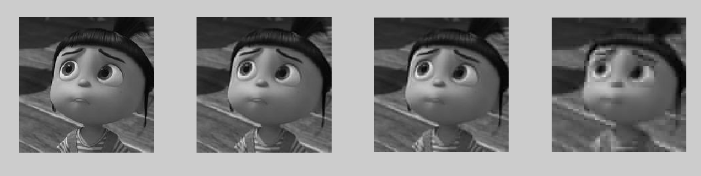展开全文• 一，背景介绍 ...高频系数一般保存的是图像的边界、纹理信息，低频信息主要是保存的图像中平坦区域信息。 二，图像 二维DCT变换就是将二维图像从空间域转换到频率域。形象的说，就是计算出图像...
一，背景介绍
DCT，即离散余弦变换，常用图像压缩算法，步骤如下
1）分割，首先将图像分割成8x8或16x16的小块；
2）DCT变换，对每个小块进行DCT变换；
3）舍弃高频系数（AC系数），保留低频信息（DC系数）。高频系数一般保存的是图像的边界、纹理信息，低频信息主要是保存的图像中平坦区域信息。
4）图像的低频和高频，高频区域指的是空域图像中突变程度大的区域（比如目标边界区域），通常的纹理丰富区域。

二，图像
二维DCT变换就是将二维图像从空间域转换到频率域。形象的说，就是计算出图像由哪些二维余弦波构成
F=AfAT$F=Af{A}^{T}$F = AfA^T
A(i,j)=c(i)cos[(j+0.5)πNi]$A\left(i,j\right)=c\left(i\right)cos\left[\frac{\left(j+0.5\right)\pi }{N}i\right]$A(i,j) = c(i) cos[\frac{(j+0.5)\pi} {N}i]

其中F就是变换得到的系数，f是图像的像素值，A是转换矩阵，其中i为二维波的水平方向频率，j为二维波的垂直方向频率，取值范围都是0-(N-1)，N是图像块的大小，
c(i)=⎧⎩⎨⎪⎪1N−−√,2N−−√,i=0i≠0$c\left(i\right)=\left\{\begin{array}{rcl}\sqrt{\frac{1}{N}},& & i=0\\ \sqrt{\frac{2}{N}},& & i\ne 0\end{array}$c(i)=\left\{
\begin{array}{rcl}
\sqrt{\frac{1}{N}}, & & {i =0}\\
\sqrt{\frac{2}{N}}, & & {i \neq{0}}\\
\end{array} \right.
1）求出转换矩阵A；
2）利用转换矩阵A，转换到频域，即由图像 f 得到系数矩阵F。

三，Matlab实现图像块DCT变换

clc;clear;
f = (rand(4,4)*100); % 生成4x4块
% 1，根据公式，生成转换矩阵A
for i=0:3
for j=0:3
if i == 0
c = sqrt(1/4);
else
c = sqrt(2/4);
end
A(i+1, j+1) = c * cos( (j + 0.5)* pi * i / 4 ); % 生成转换矩阵
end
end

% 2，利用转换矩阵A，进行转换
dct_my = A*f*A'; % 转换
dct_matlab = dct2(f); % matlab自带函数转换


结果：


f =

89.0903   14.9294   81.4285   19.6595
95.9291   25.7508   24.3525   25.1084
54.7216   84.0717   92.9264   61.6045
13.8624   25.4282   34.9984   47.3289

dct_my =

197.7977   21.3312    5.8547   40.7995
10.7399   48.3374   21.6041   46.3630
-34.4348  -18.4205    0.7236   18.6270
51.2061  -20.9533  -41.4148    8.2377

dct_matlab =

197.7977   21.3312    5.8547   40.7995
10.7399   48.3374   21.6041   46.3630
-34.4348  -18.4205    0.7236   18.6270
51.2061  -20.9533  -41.4148    8.2377

>> 

四，DCT反变换
F=AfAT$F=Af{A}^{T}$F=AfA^T
f=A−1F(AT)−1$f={A}^{-1}F\left({A}^{T}{\right)}^{-1}$f = A^{-1} F (A^T)^{-1}
A是正交矩阵，所以有AT=A−1${A}^{T}={A}^{-1}$$A^T=A^{-1}$,所以求得：
f=ATFA$f={A}^{T}FA$f = A^TFA
五，Matlab实现DCT反变换
基于前面得到的转换矩阵A，则DCT反转换后面加一行代码即可：f_convert=A′FA$f\mathrm{_}convert={A}^{\prime }FA$$f\_convert = A'FA$

clc;clear;
f = (rand(4,4)*100)； % 生成4x4块
for i=0:3
for j=0:3
if i == 0
c = sqrt(1/4);
else
c = sqrt(2/4);
end
A(i+1, j+1) = c * cos( (j + 0.5)* pi * i / 4 );
end
end

dct_my = A*f*A'；
dct_matlab = dct2(f)；

f_convert = A'*dct_my*A；



f =

22.8977   53.8342   10.6653   81.7303
91.3337   99.6135   96.1898   86.8695
15.2378    7.8176    0.4634    8.4436
82.5817   44.2678   77.4910   39.9783

dct_my =

204.8538    1.1802    9.6825   -7.4417
21.7177  -30.4859    8.3810  -50.0097
1.8694   -9.0912   10.7823   -3.4473
-121.8989  -10.6487   16.1003  -22.1974

dct_matlab =

204.8538    1.1802    9.6825   -7.4417
21.7177  -30.4859    8.3810  -50.0097
1.8694   -9.0912   10.7823   -3.4473
-121.8989  -10.6487   16.1003  -22.1974

f_convert =

22.8977   53.8342   10.6653   81.7303
91.3337   99.6135   96.1898   86.8695
15.2378    7.8176    0.4634    8.4436
82.5817   44.2678   77.4910   39.9783

>> 

六，对图像进行DCT变换
效果图，分别是原图，所有dct系数小块组成图，反dct变换回来的图：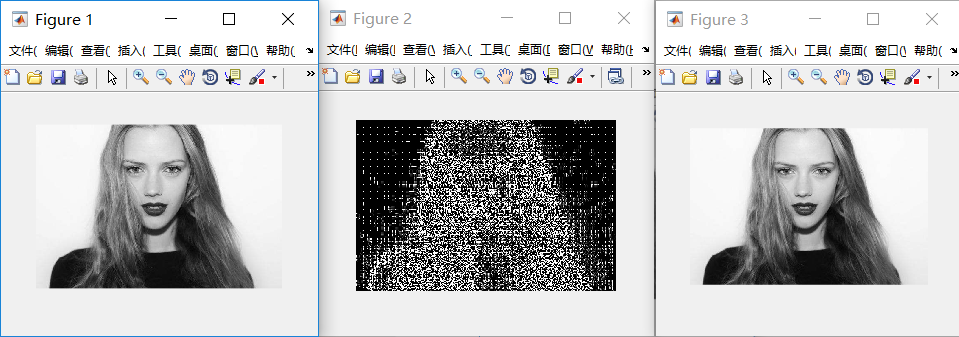将图像分成8x8的小块，对每个小块依次进行dct变换，反变换回来时，也是依次处理每个小块。
matlab代码：

clc;clear;
figure, imshow(img);

% 1，使图像行列为 8的倍数
[row,col] = size(img);
row = round(row/8) * 8;
col = round(col/8) * 8;
img = imresize(img, [row, col]);

% 2，对图像块进行dct变换
img_dct = zeros(row, col); % 存放转换后的dct系数
for i=1:8:row-7
for j=1:8:col-7
img_block = img(i:i+7, j:j+7);
dct_block = dct2(img_block); % 也可用刚才实现的(定义成一个函数即可)
% imshow(dct_block); % 显示dct块
img_dct(i:i+7, j:j+7) = dct_block;
end
end
figure, imshow(img_dct); % 显示生成的dct系数

% 3，dct反变换
new_img = zeros(row,col);
for i=1:8:row-7
for j=1:8:col-7
dct_block = img_dct(i:i+7, j:j+7);
img_block = idct2(dct_block); % 也可用刚才实现的(定义成一个函数即可)
new_img(i:i+7, j:j+7) = img_block;
end
end
figure,  imshow(mat2gray(new_img)); % 显示反变换回来的图像


放大后的dct系数块组成的图像：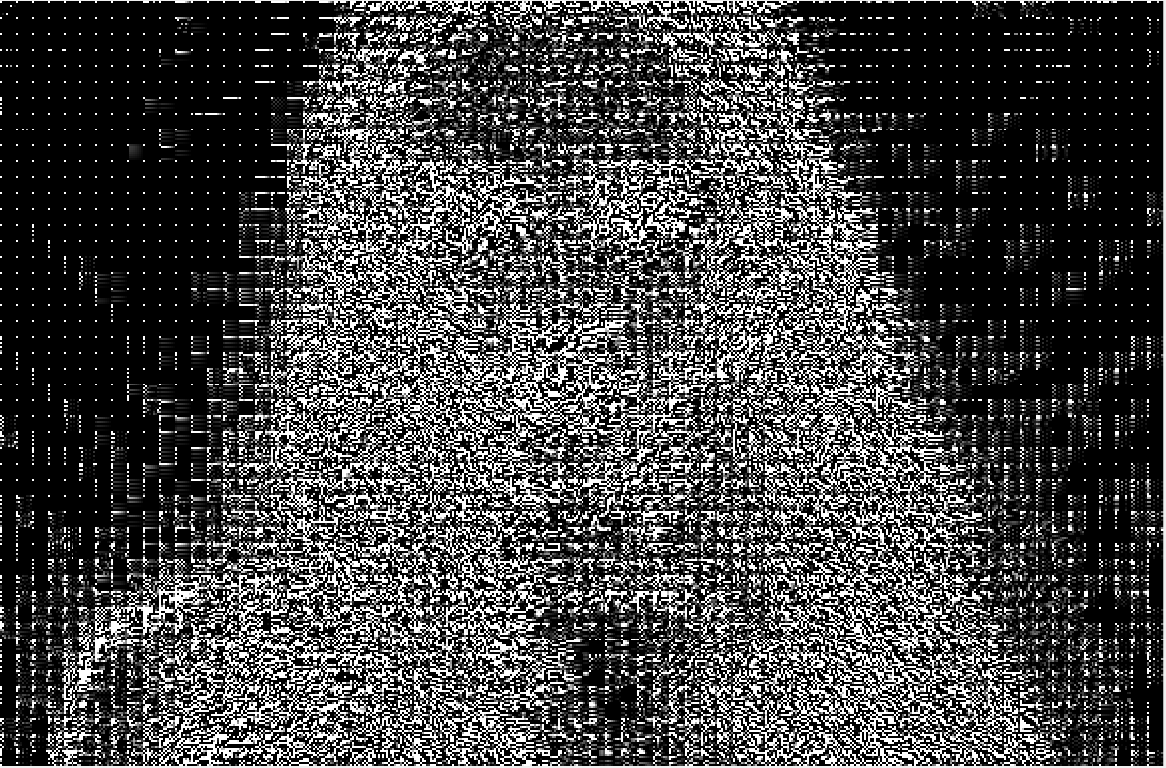1）发现每个小块的左上角，即一个DC系数，最亮，保存的是原图像低频信息，反应的是空域图像中平坦区域的信息；
2）小块的其他地方，即63个AC系数，保存的是高频信息，反应的是空域图像中的突变区域的信息；
3）对整个图像而言，背景区域是平坦区域，没有纹理信息，所以AC系数很小，而代表亮度信息的DC系数很大；
4）头发区域不仅含有亮度信息，纹理信息也丰富，所以AC系数值很大。

七，不分块，直接对整个图像DCT变换

clc;clear;
figure, imshow(img);

% 1，对整个图像dct变换
dct_img = dct2(img);
figure, imshow(log(abs(dct_img)), colormap(gray(5)));
colorbar;

% 2，量化, 使得矩阵中小于0.1的值置为0，变得稀疏
dct_img(abs(dct_img)<0.1)=0;

% 3，反变换回来
new_img = idct2(dct_img);
new_img = mat2gray(new_img);
figure, imshow(new_img);  

效果图，分别是原图、整个图像变换后系数图、反变换得到的图：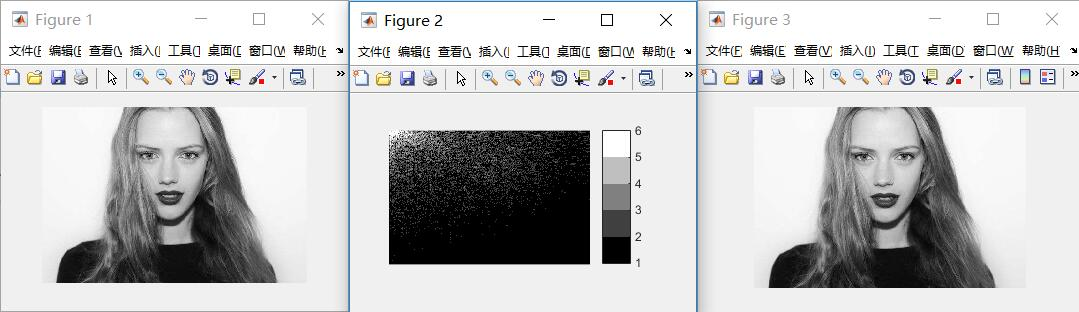展开全文• 1DCT变换的学习笔记 DCT变换是一种可逆的变化，也就是说：DCT变换是一种一一映射；
1 DCT变换的学习笔记

DCT变换是一种可逆的变化，也就是说：DCT变换是一种一一映射；

展开全文DCT变换
• RGB=imread('原始图像'); GR=rgb2gray(RGB);%转换成灰度图像 figure(1);imshow(GR); D=dct2(GR); %计算DCT figure(2);imshow(log(abs(D)),[]); colormap(gray(4));colorbar; D(abs(D) I=idct2...
• 以下是原创的C++代码，可在VC6.0及以上版本测试，相关头文件若缺失请在百度或谷歌下载。 #include <stdio.h> #include "BmpRot.h" #include "stdlib.h" #include "math.h" #include <...DCT图像压缩 图像处理 DCT变换
• 这是一个c编写的有关图像处理的小程序，里面主要对图像进行dct变换，以及反变换。图像dct变换
• 计算图像的2维离散余弦变换，将其中数值最小的10%、30%……个系数设定为0，作反变换看它们与原始图像的区别，并计算均方根误差 重点： 实现将数值最小的10%个系数设定为0的步骤： 1.将矩阵排成一个向量，并利用sort...
• 2015年10月13日 18:37:21 及时澍雨Timely 阅读数：12415 标签： 图像处理 DCT 二维 余弦变换 频率域 更多 个人分类： Image Processing 原文为http://blog.csdn.net/lvhao92/article/details/49099129 DCT变换...
• 图像DCT算法 @(Learning)[Auspice Vinson] 介绍 DCT 离散余弦变换，常用图像变换算法 分割：将图像分割成88或1616的小块 DCT变换：对每块进行DCT变换 舍弃高频系数(AC系数)，保留低频系数(DC系数) 高频系数一般...算法 计算机视觉
• DCT变换、DCT反变换、分块DCT变换 原文 http://www.cnblogs.com/lzhen/p/3947600.html 一、引言  DCT变换的全称是离散余弦变换(Discrete Cosine Transform)，主要用于将数据或图像的压缩，能够将空域的信号转换...
• 在网上收集的关于DCT的资料，以及改进的DCT。有用的人下吧
• 本代码利用matlab实现数字图像水印，包含了加密，解密（属于不需要原图即可获得水印图像的类型）。也包含了高斯滤波，白噪声，剪切，旋转等攻击。matlab 多媒体技术 图像处理图像处理